# Name the acids illustrated below. a. b . c. d. e.### Chemistry: An Atoms First Approach

2nd Edition
Steven S. Zumdahl + 1 other
Publisher: Cengage Learning
ISBN: 9781305079243

#### Solutions

Chapter
Section### Chemistry: An Atoms First Approach

2nd Edition
Steven S. Zumdahl + 1 other
Publisher: Cengage Learning
ISBN: 9781305079243
Chapter 3, Problem 121E
Textbook Problem
85 views

## Name the acids illustrated below.a.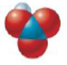b.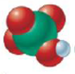c.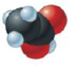d.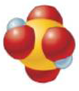e.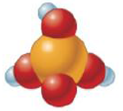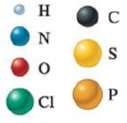(a)

Interpretation Introduction

Interpretation: The name of the binary compound formed from the given structures and compound is to be stated.

Concept introduction: The oxidation state of an element corresponds to the group number of that element. In case of non-metals, the oxidation state can be calculated as groupnumber-8. While naming an ionic compound, the cation is named first followed by the naming of the anion. If the cation exhibits more than one oxidation state then the current oxidation state of the cation is to be mentioned.

To determine: The naming of given structure

### Explanation of Solution

The given structure of (HNO3) is

Figure 1

Rules for binary compound:

• In naming of binary compound, the name of cation comes first which is the name of element. Anion is named second which is also the name of element. Monatomic anions are named with the ending -ide.
• Roman numerals are used to show the oxidation state because some elements exhibit more than one oxidation state.
• When oxygen is not present in anion, then acids is named by using “hydro-” in prefix.
• If the name of anions ends in “ate-” then acid name ends in –ic or –ric
• If the name of anion ends in “-ite”, then the name of acid ends in –ous

(b)

Interpretation Introduction

Interpretation: The name of the binary compound formed from the given structures and compound is to be stated.

Concept introduction: The oxidation state of an element corresponds to the group number of that element. In case of non-metals, the oxidation state can be calculated as groupnumber-8. While naming an ionic compound, the cation is named first followed by the naming of the anion. If the cation exhibits more than one oxidation state then the current oxidation state of the cation is to be mentioned.

To determine: The  naming of given structure.

(c)

Interpretation Introduction

Interpretation: The name of the binary compound formed from the given structures and compound is to be stated.

Concept introduction: The oxidation state of an element corresponds to the group number of that element. In case of non-metals, the oxidation state can be calculated as groupnumber-8. While naming an ionic compound, the cation is named first followed by the naming of the anion. If the cation exhibits more than one oxidation state then the current oxidation state of the cation is to be mentioned.

To determine: The  naming of given structure.

(d)

Interpretation Introduction

Interpretation: The name of the binary compound formed from the given structures and compound is to be stated.

Concept introduction: The oxidation state of an element corresponds to the group number of that element. In case of non-metals, the oxidation state can be calculated as groupnumber-8. While naming an ionic compound, the cation is named first followed by the naming of the anion. If the cation exhibits more than one oxidation state then the current oxidation state of the cation is to be mentioned.

To determine: The  naming of given structure.

(e)

Interpretation Introduction

Interpretation: The name of the binary compound formed from the given structures and compound is to be stated.

Concept introduction: The oxidation state of an element corresponds to the group number of that element. In case of non-metals, the oxidation state can be calculated as groupnumber-8. While naming an ionic compound, the cation is named first followed by the naming of the anion. If the cation exhibits more than one oxidation state then the current oxidation state of the cation is to be mentioned.

To determine: The  naming of given structure.

### Still sussing out bartleby?

Check out a sample textbook solution.

See a sample solution

#### The Solution to Your Study Problems

Bartleby provides explanations to thousands of textbook problems written by our experts, many with advanced degrees!

Get Started

Find more solutions based on key concepts
Name the following compounds. a Na2SO4 b Na3N c CuCl d Cr2O3

General Chemistry - Standalone book (MindTap Course List)

Just 3 ounces of salmon, a naturally rich source of vitamin D, provides most of an adult's daily need for vitam...

Nutrition: Concepts and Controversies - Standalone book (MindTap Course List)

What is the primary function of the electron transport chain?

Chemistry for Today: General, Organic, and Biochemistry

What is the defining feature of a synovial joint?

Human Biology (MindTap Course List)

A sphere of radius R = 1.00 m surrounds a particle with charge Q = 50.0 C located at its center as shown in Fig...

Physics for Scientists and Engineers, Technology Update (No access codes included)

What is meant by overfishing? Are most of the worlds marine fishes overfished?

Oceanography: An Invitation To Marine Science, Loose-leaf Versin

If two stars differ by 8 magnitudes, what is their flux ratio?

Foundations of Astronomy (MindTap Course List)